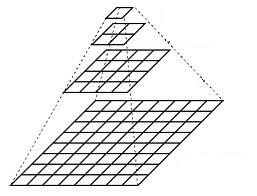## 模板匹配简介$$d_{f,t}^2(u,v)=\sum_{x,y}[f(x,y)-t(x-u,y-u)]^2$$

$$d_{f,t}^2(u,v)=\sum_{x,y}(f^2(x,y) - 2f(x,y)t(x-u,y-u) + t^2(x-u,y-v))$$

$$c(u,v) = \sum_{x,y}f(x,y)t(x-u,y-u)$$

$$\gamma(u,v) = \frac{\sum_{x,y}[f(x,y)-\overline{f}_{u,v}][t(x-u,y-v)-\overline{t}]}{\sqrt{\sum_{x,y}[f(x,y)-\overline{f}_{u,v}]^2[t(x-u,y-v)-\overline{t}]^2}}$$

## NCC算法实现

##### 快速傅里叶变换计算卷积

$$分子 = \sum_{x,y}f’(x,y)t(x-u,y-v)$$

$$分母 = \sqrt{\sum_{x,y}[t(x-u,y-v)-\overline{t}]^2[f^2(x,y)-\overline{f}_{u,v}^2]}$$
(推倒过程并不复杂，略去)

//快速傅里叶变换计算卷积，结果存放在result中
crossCorr( img, templ, result, result.size(), result.type(), Point(0,0), 0, 0);

if( method == CV_TM_CCORR )
return;

double invArea = 1./((double)templ.rows * templ.cols);
double invArea = 1./((double)templ.rows * templ.cols);

Mat sum, sqsum;
Scalar templMean, templSdv;
double *q0 = 0, *q1 = 0, *q2 = 0, *q3 = 0;
double templNorm = 0, templSum2 = 0;

if( method == CV_TM_CCOEFF )
{
integral(img, sum, CV_64F);
templMean = mean(templ);
}
else//以下是NCC实现，支持彩色图像
{
integral(img, sum, sqsum, CV_64F);//计算积分图像，sum为f(x,y)积分图，sqsum为f^2(x,y)积分图
meanStdDev( templ, templMean, templSdv );

//模板的方差，用来计算分母中的(1)
templNorm = templSdv*templSdv + templSdv*templSdv + templSdv*templSdv + templSdv*templSdv;

if( templNorm < DBL_EPSILON && method == CV_TM_CCOEFF_NORMED )
{
result = Scalar::all(1);
return;
}

templSum2 = templNorm + templMean*templMean + templMean*templMean + templMean*templMean + templMean*templMean;

if( numType != 1 )
{
templMean = Scalar::all(0);
templNorm = templSum2;
}

templSum2 /= invArea;
templNorm = std::sqrt(templNorm);
templNorm /= std::sqrt(invArea); // care of accuracy here

q0 = (double*)sqsum.data;
q1 = q0 + templ.cols*cn;  // The first row and column of the integral image are all zeros
q2 = (double*)(sqsum.data + templ.rows*sqsum.step);
q3 = q2 + templ.cols*cn;
}

double* p0 = (double*)sum.data;
double* p1 = p0 + templ.cols*cn;
double* p2 = (double*)(sum.data + templ.rows*sum.step);
double* p3 = p2 + templ.cols*cn;

int sumstep = sum.data ? (int)(sum.step / sizeof(double)) : 0;
int sqstep = sqsum.data ? (int)(sqsum.step / sizeof(double)) : 0;

int i, j, k;

for( i = 0; i < result.rows; i++ )
{
float* rrow = result.ptr<float>(i);
int idx = i * sumstep;
int idx2 = i * sqstep;

for( j = 0; j < result.cols; j++, idx += cn, idx2 += cn )
{
double num = rrow[j], t;
double wndMean2 = 0, wndSum2 = 0;

if( numType == 1 )
{
for( k = 0; k < cn; k++ )
{
t = p0[idx+k] - p1[idx+k] - p2[idx+k] + p3[idx+k];
wndMean2 += t*t;
num -= t*templMean[k];
}

wndMean2 *= invArea;
}

if( isNormed || numType == 2 )
{
for( k = 0; k < cn; k++ )
{
t = q0[idx2+k] - q1[idx2+k] - q2[idx2+k] + q3[idx2+k];
wndSum2 += t;
}

if( numType == 2 )
{
num = wndSum2 - 2*num + templSum2;
num = MAX(num, 0.);
}
}

if( isNormed )
{
t = std::sqrt(MAX(wndSum2 - wndMean2,0))*templNorm;//分母项结果
if( fabs(num) < t )
num /= t;
else if( fabs(num) < t*1.125 )
num = num > 0 ? 1 : -1;
else
num = method != CV_TM_SQDIFF_NORMED ? 0 : 1;
}

rrow[j] = (float)num;
}
}

##### 图像金字塔##### End

NCC是基于图像灰度的一种匹配技术，在大部分情况下都可以适用。但是一定程度下还是会受光照不均匀影响，另外对于目标物体存在混乱，遮挡等情况匹配效果十分不理想。下面有机会的话会介绍基于边缘的模板匹配。

PS：本文适合有一定图像处理基础的人阅读，由于公式编辑实在麻烦，省去部分推倒过程。